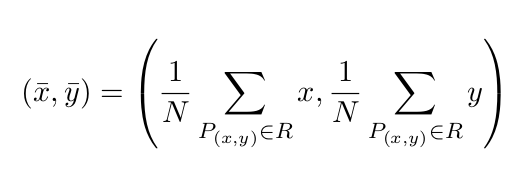# Department of Mathematics and Computer Science

www.univ-soukahras.dz/en/dept/mi

Module: Initiation à la recherché et code de déontologie

1. Information
2. Questions

## Writing Equations using Latex (3)

 1 votes What's the latex code for the following Equation:Asked on 19:28, Monday 16 May 2016 By Imed BOUCHRIKA In Initiation à la recherché et code de déontologie

 1 votes \begin{equation} (\bar{x},\bar{y}) = (\frac{1}{N} \; \sum_{P_{(x,y) \in R }} \; x, \frac{1}{N} \; \sum_{P_{(x,y) \in R }} \; y ) \end{equation} Answered on 21:55, Tuesday 17 May 2016 by Hanen Rouainia(322 points) In Initiation à la recherché et code de déontologie

 0 votes you should insert a big parenthesis hanen \begin{equation}     (\bar{x},\bar{y}) =  \bigg(                                          \frac{1}{N} \sum_{P_{(x,y)} \in R} X , \frac{1}{N} \sum_{P_{(x,y)} in R} Y                                     \bigg) \end{equation} Answered on 23:17, Wednesday 25 May 2016 by mohammed tebib(377 points) In Initiation à la recherché et code de déontologie
 0 votes \begin{equation} (\bar{x},\bar{y}) = \bigg ( \frac{1}{N} \sum_{P_{(x,y) \in R}} X,\frac{1}{N} \sum_{P_{(x,y) \in R}} y \bigg ) \end{equation} Answered on 20:56, Friday 27 May 2016 by amirat abdallah(257 points) In Initiation à la recherché et code de déontologie